Language :

Expected Quantitative Aptitude Questions for SBI Mains 2018Question 1 The ratio of difference between CI and SI on a sum with the same rate of interest for 3 years and CI and SI for 2 years on the same sum with the same rate of interest is 33: 10. Find rate of interest.  The ratio of difference between CI and SI on a sum with the same rate of interest for 3 years and CI and SI for 2 years on the same sum with the same rate of interest is 33: 10. Find rate of interest. Read Less
 A 15%B 30%C 10%D 20%E 25%
 Question 2 A sum of money invested at 20% rate compounded annually would have fetched Rs. 246 more in one year if CI was compounded half yearly. Find the sum of money invested.  A sum of money invested at 20% rate compounded annually would have fetched Rs. 246 more in one year if CI was compounded half yearly. Find the sum of money invested. Read Less
 A Rs.21400B Rs.24600C Rs.28500D Rs.25400E Rs.22500
 Question 3 A sum of Rs. 125 becomes Rs. 343 in 3 years. Find the rate of interest if it is compounded annually.  A sum of Rs. 125 becomes Rs. 343 in 3 years. Find the rate of interest if it is compounded annually. Read Less
 A 30%B 20%C 25%D 40%E 50%
 Question 4 A sum amounts to Rs. 8000 in 3 years and Rs. 27000 in 6 years when compounded annually. Find the rate of interest.  A sum amounts to Rs. 8000 in 3 years and Rs. 27000 in 6 years when compounded annually. Find the rate of interest. Read Less
 A 30%B 25%C 50%D 40%E 20%
 Question 5 Find the difference between compound interest calculated half yearly and simple interest calculated per annum on a sum of Rs. 7000 at 20% rate of interest after one and half years. Find the difference between compound interest calculated half yearly and simple interest calculated per annum on a sum of Rs. 7000 at 20% rate of interest after one and half years.Read Less
 A Rs. 323B Rs. 217C Rs. 523D Rs. 417E Rs. 227
 Question 6 63% of 210 + 47% of 310 – 30% of 175 = ?   63% of 210 + 47% of 310 – 30% of 175 = ?  Read Less
 A 224B 225.5C 220D 227.5E 228.5
 Question 7 ? of 3/7 – 42% of 250 = 315   ? of 3/7 – 42% of 250 = 315  Read Less
 A 970B 1000C 980D 985E 975
 Question 8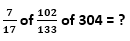Read Less
 A 92B 88C 94D 96E 98
 Question 9 341.46 + 273.43 + 583.11 – 881 = ?  341.46 + 273.43 + 583.11 – 881 = ? Read Less
 A 321B 317C 319D 313E 314
 Question 10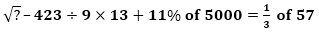Read Less
 A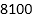B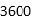C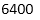D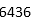E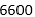## SBI Clerk - ONLINE TEST

IBT Institute offer you the most trusted and highly recommended Test Series for SBI Clerk Exam. 8 out of every 10 candidates selected in SBI Clerk last year opted for IBT Online Test Series.

## SBI Clerk - CLASS ROOM COURSE

Keeping in mind the SBI Clerk Exam, IBT has launched Special batches for SBI Clerk Exam to guide you through your preparations. Seats filling fast. Don’t miss out!!Correspondence Course

For SBI PO Exam (Pre+Main)

9 Books , 350 Tests

52 Assignments , Online Doubts